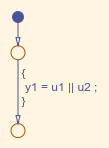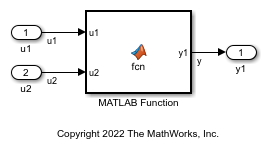Main Content

# Relational and Logical Operators

This example shows how to implement relational and logical operators by using Simulink blocks, Stateflow Charts, and MATLAB Function blocks.

### Modeling Pattern for Relational or Logical Operators — Simulink Blocks

To include a logical operation in your model, use the Logical Operator block from the Logic and Bit Operations library.

1. Open example model `ex_data_type_SL`.The Logical Operator block performs an OR operation in the model. To change the operation, double-click the block and set the Operator field to any of the operations in the menu.

You can implement relational operators by replacing the Logical Operator block with a Relational Operator block.

2. To build the model and generate code, press Ctrl+B.

The code implementing the logical operator OR is in the `ex_logical_SL_step` function in `ex_logical_SL.c`.

```/* Exported block signals */ boolean_T u1; /* '<Root>/u1' */ boolean_T u2; /* '<Root>/u2' */ boolean_T y1; /* '<Root>/y1' */ /* Model step function */ void ex_logical_SL_step(void) { /* Outport: '<Root>/y1' incorporates: * Inport: '<Root>/u1' * Inport: '<Root>/u2' * Logic: '<Root>/Logical Operator' */ y1 = (u1 || u2); } ```

### Modeling Pattern for Relational and Logical Operators — Stateflow Chart

1. Open example model `ex_data_type_SF`.In the Stateflow chart, the relational or logical operation actions are on the transition from one junction to another. Relational statements specify conditions to conditionally allow a transition. In that case, the statements are within square brackets.

2. To build the model and generate code, press Ctrl+B.

The code implementing the logical operator OR is in the `ex_logical_SF_step` function in `ex_logical_SF.c`.

```/* Exported block signals */ boolean_T u1; /* '<Root>/u1' */ boolean_T u2; /* '<Root>/u2' */ boolean_T y1; /* '<Root>/Logical Operator' */ /* Model step function */ void ex_logical_SF_step(void) { /* Chart: '<Root>/Logical Operator' incorporates: * Inport: '<Root>/u1' * Inport: '<Root>/u2' */ y1 = (u1 || u2); } ```

### Modeling Pattern for Relational and Logical Operators — MATLAB Function Block

This example shows the MATLAB Function block method for incorporating operators into the generated code by using a relational operator.

1. Open example model `ex_logical_ML`.2. The MATLAB Function Block contains this function:

```function y1 = fcn(u1, u2) y1 = u1 > u2; end ```

3. To build the model and generate code, press Ctrl+B.

The generated code appears in `ex_data_type_ML.c`:

```/* Exported block signals */ real_T u1; /* '<Root>/u1' */ real_T u2; /* '<Root>/u2' */ boolean_T y; /* '<Root>/MATLAB Function' */ /* Model step function */ void ex_logical_ML_step(void) { /* MATLAB Function: '<Root>/MATLAB Function' incorporates: * Inport: '<Root>/u1' * Inport: '<Root>/u2' */ y = (u1 > u2); } ```

Download guide